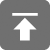# 工学1号馆

home

## C语言指针传递详解

Wu Yudong    June 27, 2015     C   335

### 用指针传递数据

#include<stdio.h>
void swap(int* a, int* b)
{
int tmp;
tmp = *a;
*a = *b;
*b = tmp;
}
int main()
{
int m, n;
m = 5;
n = 10;
printf("m=%d, n=%d\n",m, n);
swap(&m, &n);
printf("m=%d, n=%d\n",m, n);
return 0;
}

### 传递指向常量的指针

#include<stdio.h>
void passconstant(const int* num1, int*num2)
{
*num2 = *num1;
}
int main()
{
const int a = 100;
int b = 5;
printf("a=%d, b=%d\n",a, b);
passconstant(&a, &b);
printf("a=%d, b=%d\n",a, b);
return 0;
}

#include<stdio.h>
void passconstant(const int* num1, int* num2)
{
*num1 = 100;
*num2 = 200;
}
int main()
{
const int limit = 100;
passconstant(&limit, &limit);
return 0;
}

### C语言中堆和栈的区别

1、栈区（stack）— 由编译器自动分配释放 ，存放函数的参数值，局部变量的值等。其操作方式类似于数据结构中的栈。

2、堆区（heap） — 一般由程序员分配释放， 若程序员不释放，程序结束时可能由OS回收 。注意它与数据结构中的堆是两回事，分配方式倒是类似于链表。

3、全局区（静态区）（static），全局变量和静态变量的存储是放在一块的，初始化的全局变量和静态变量在一块区域， 未初始化的全局变量和未初始化的静态变量在相邻的另一块区域。程序结束后由系统释放。

4、文字常量区 —常量字符串就是放在这里的， 程序结束后由系统释放

5、程序代码区—存放函数体的二进制代码。内存中的栈区处于相对较高的地址以地址的增长方向为上的话，栈地址是向下增长的，栈中分配局部变量空间，堆区是向上增长的用于分配程序员申请的内存空间。另外还有静态区是分配静态变量，全局变量空间的；只读区是分配常量和程序代码空间的；以及其他一些分区。

#include<stdio.h>
#include<stdlib.h>
int a = 0; //全局初始化区
char *p1; //全局未初始化区
int main()
{
int b; //栈
char s[] = "abc"; //栈
char *p2; //栈
char *p3 = "123456"; //123456\0在常量区，p3在栈上。
static int c =0; //全局（静态）初始化区
p1 = (char*)malloc(10);  //堆
p2 = (char*)malloc(10);
return 0;
}

1.申请后系统的响应

2.申请效率的比较

3.申请大小的限制

4.堆和栈中的存储内容

### 局部变量指针

#include<stdio.h>
#include<stdlib.h>
int* allocateArray(int size, int value)
{
int arr[size];
for(int i = 0; i < size; i++) {
arr[i] = value;
}
return arr;
}
int main()
{
int* vector = allocateArray(5, 45);
for(int i = 0; i < 5; i++) {
printf("%d\n", vector[i]);
}
return 0;
}

#include<stdio.h>
#include<stdlib.h>
int* allocateArray(int size, int value)
{
static int arr;
for(int i = 0; i < size; i++) {
arr[i] = value;
}
return arr;
}
int main()
{
int* vector = allocateArray(5, 45);
for(int i = 0; i < 5; i++) {
printf("%d\n", vector[i]);
}
return 0;
}

### 返回指针

• 使用malloc在函数内部分配内存并返回其地址，调用者负责释放返回的内存
• 传递一个对象给函数，让函数修改它，这样分配和释放对象的内存都是调用者的责任
#include<stdio.h>
#include<stdlib.h>
int* allocateArray(int size, int value)
{
int* arr  = (int*)malloc(size * sizeof(int));
for(int i = 0; i < size; i++) {
arr[i] = value;
}
return arr;
}
int main()
{
int* vector = allocateArray(5, 45);
for(int i = 0; i < 5; i++) {
printf("%d\n", vector[i]);
}
free(vector);
return 0;
}

#include<stdio.h>
#include<stdlib.h>
int* allocateArray(int *arr, int size, int value)
{
if(arr != NULL) {
for(int i = 0; i < size; i++) {
arr[i] = value;
}
}
return arr;
}
int main()
{
int* vector = (int*)malloc(5 * sizeof(int));
allocateArray(vector, 5, 45);
for(int i = 0; i < 5; i++) {
printf("%d\n", vector[i]);
}
free(vector);
return 0;
}

### 传递指针的指针

#include<stdio.h>
#include<stdlib.h>
void allocateArray(int **arr, int size, int value)
{
*arr = (int*)malloc(size * sizeof(int));
if(arr != NULL) {
for(int i = 0; i < size; i++) {
*(*arr + i) = value;
}
}
}
int main()
{
int* vector = NULL;
allocateArray(&vector, 5, 45);
for(int i = 0; i < 5; i++) {
printf("%d\n", vector[i]);
}
free(vector);
return 0;
}

### 二叉树递归实现与二重指针

1、二叉树的定义

typedef struct Node
{
char ch;
struct Node *lchild,*rchild;
}Node,*BTree;

Node一般可用来定义二叉树节点，而*BTree可用来定义指向二叉树（根节点）的指针

2、内存动态分配

3、递归调用

void CreateTree(Node** pTree)
{
char ch;
scanf("%c",&ch);
if(chr == '#') {
(*pTree) = NULL;
} else {
if(!((*pTree) = (Node*)malloc(sizeof(Node)))) {
exit(OVERFLOW);
}
(*pTree)->ch = chr;
CreateTree(&((*pTree)->lchild));
CreateTree(&((*pTree)->rchild));
}
}

### 参考资料：

《C专家编程》

《C和指针》